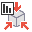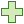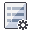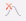Join one of our upcoming courses taking place around the world! Explore now

# Probabilistic Geometry, Stresses, and Rock Mass Strength

To define the variability of general Geometry, Stresses, and Rock Mass Strength properties:

1. Select Geometry and Stressfrom the Statistics menu.
2. To set an input parameter as a random variable in the Geometry and Stress Statistics dialog:
1. Select Addbutton to populate the next available statistical variable.
2. Under the Property column, select the desired variable from the dropdown. The Mean value set in the Input Data dialog is automatically populated.
3. Under the Distribution column, select the desired statistical distribution from the dropdown. The default Distribution = Normal.
4. Edit or populate the Mean, Std. Dev., Rel. Min, and Rel. Max columns.
5. Select OK to save the statistics.

Alternatively, to define random variables:

1. Select Input Datafrom the toolbar or Analysis menu.
2. Select the statisticsbutton beside the edit box.
3. Choose a distribution, and enter the standard deviation, relative minimum and relative maximum (this is handy for quickly defining or editing statistics for individual variables).

## Geometry

If you are performing a Probabilistic analysis with CPillar, the following Geometry, Abutment Orientation, and Unit Weight parameters can be defined as random variables:

• Pillar Length (Rectangular Pillar only)
• Pillar Width (Rectangular Pillar only)
• Pillar Height
• Abutment Plunge (Rigid analysis only)
• Abutment Trend (Rigid analysis only)
• Rock Unit Weight
• Overburden Unit Weight
• Facedip (Voussoir analysis only)

## Stresses

For a Probabilistic analysis using the Rigid and Elastic analyses you can define the following Lateral Stress, Overburden, and Water parameters can be defined as random variables:

• Locked In Stress
• K1 (or Kx for Rectangular Pillar)
• K3 (or Ky for Rectangular Pillar)
• Trend 3
• Sigma 1 (or Sigma x for Rectangular Pillar)
• Sigma 3 (or Sigma y for Rectangular Pillar)
• Overburden Thickness
• Overbuden Unit Weight
• Water Height
• Water Unit Weight

### Voussoir Analysis

For a Probabilistic analysis using the Voussoir analysis type you can define the following Lateral Stress, and Overburden parameters as random variables:

• Overburden Thickness
• Overburden Unit Weight
• Support Pressure

## Rock Mass Strength

If you are performing a Probabilistic analysis with CPillar, you can define the following Rock Mass Strength parameters as random variables:

• Intact UCS
• Rock Mass Modulus (Elastic and Voussoir only)
• Poisson Ratio (Voussoir only)